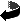### Basic Volts, Ohms, Amps, and Power Problems

1. A circuit that draws 825 ma from an 18V source repesents a load of _________ ohms.
2. A 115Vac generator must be able to supply ___ amps into a 230 ohm load.
3. A 12A current through a 3.5 ohm resistance will develop ___ V across the resistance.
4. A resistance of 390 ohms, in parallel with a 1.2K ohm resistance, will represent a total of _____ ohms.
5. A resistance of 510 ohms, in series with 2.7K ohms, will represent a total of ______ ohms.
6. The wattage dissapated by the combination of a 1.2K ohm resistance, in series with a 750 ohm resistance, with 85V applied across that series resistance combination, would be _______ watts total.
7. A circuit that is drawing 4.5A, with 115Vac across that circuit, will result in a usage of ____ Kilo-Watt-Hours, over a 35 hour time frame.
8. If the voltage across a 39K ohm resistor is doubled the power dissipated by the resistor will (double/triple/quadrouple).
9. If the current through a 2.2K ohm resistance is tripled, the power dissipated from the resistance will be ___ times more.
10. If a 1.8K ohm resistance, which is series with a 470 ohm resistance, has a voltage drop of 5.12V across the 1.8K ohm resistance, then ______ V should be expected across the 470 ohm resistance.
11. If a 3.9K ohm resistance, which is in parallel with a 5.6K ohm resistance, has 1.16ma flowing through the 3.9K ohm, then ________ ma should be expected to be flowing through the 5.6K ohm resistance.
12. A circuit that draws 750ma from a 12V source represents a load of _______ ohms
13. A 48V generator must be able to supply ______ amps into a 250 ohm load.
14. A 8.55A current through a 35 ohm resistance will develop ___ V across the resistance.
15. A resistance of 470 ohms, in parallel with a 1.8K ohm resistance, will represent a total of _____ ohms.
16. A resistance of 220 ohms, in series with 2.2K ohms, will represent a total of ______ ohms.
17. A circuit that is drawing 35A, with 115Vac across that circuit, will result in a usage of ____ Kilo-Watt-Hours, over a 12 hour time frame.
18. If a 1200 ohm resistance, which is series with a 510 ohm resistance, has a voltage drop of 4.85V across the 1200 ohm resistance, then ______ V should be expected across the 510 ohm resistance.
19. If a 4.7K ohm resistance, which is in parallel with a 6.8K ohm resistance, has 2.15ma flowing through the 4.7K ohm, then ________ ma should be expected to be flowing through the 6.8K ohm resistance.Return to Main Page

BasicWrd1FB.html - SfE-DCS, ddf - 07/28/2001